# PSAT Math : How to find arithmetic mean

## Example Questions

← Previous 1 3 4 5 6

### Example Question #1 : Data Analysis

This semester, Mary had five quizzes that were each worth 10% of her grade. She scored 89, 74, 84, 92, and 90 on those five quizzes. Mary also scored a 92 on her midterm that was worth 25% of her grade, and a 91 on her final that was also worth 25% of her class grade. What was Mary's final grade in the class?

85

93

89

87

91

89

Explanation:

To find her average grade for the class, we need to multiply Mary's test scores by their corresponding weights and then add them up.

The five quizzes were each worth 10%, or 0.1, of her grade, and the midterm and final were both worth 25%, or 0.25.

average = (0.1 * 89) + (0.1 * 74) + (0.1 * 84) + (0.1 * 92) + (0.1 * 90) + (0.25 * 92) + (0.25 * 91) = 88.95 = 89.

Looking at the answer choices, they are all spaced 2 percentage points apart, so clearly the closest answer choice to 88.95 is 89.

### Example Question #1 : How To Find Arithmetic Mean

A, B, C, D, and E are integers such that A < B < C < D < E. If B is the average of A and C, and D is the average of C and E, what is the average of B and D?

(A + 2C + E)/4

(E)/2

(2A + C + 2E)/2

(A + 2C + E)/2

(A + E)/4

(A + 2C + E)/4

Explanation:

The average of two numbers can be calculated as the sum of those numbers divided by 2. B would thus be calculated as (C)/2, and D would be calculated as (E)/2. To find the average of those values, you would add them up and divide by 2: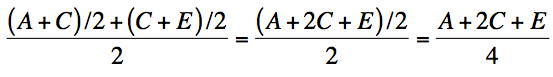### Example Question #2 : Arithmetic Mean

The average (arithmetic mean) of m, n and p is 8. If m + n = 15 then p equals:

8

24

7.5

15

9

9

Explanation:

If the arithmetic mean of the three numbers is 8, then the three numbers total 24. We are given m + n, leaving p to equal 24 – 15 = 9.

### Example Question #1 : Statistics

It takes Johnny 25 minutes to run a loop around the track. He runs a second loop and it takes him 30 minutes. If the track is 5.5 miles long, what is his average speed in miles per hour?

10

11

13

12

12

Explanation:

The minutes must be converted to hours which gives 11/12 hours. The total distance he runs is 11 miles. 11/(11/12) = 12.

### Example Question #1 : Statistics

I recently joined a bowling team. Each night we play three games. During my first two games I scored a 112 and 134, what must I score on my next game to ensure my average for that night will be a 132?

140

175

150

132

150

Explanation:

To find the average you add all the games and divide by the number of games. In this case we have 112 + 134 + x = 246 + x. If we divide by 3 and set our answer to 132, we can solve for x by cross multiplying and solving algebraically. We can also solve this problem using substitution.

### Example Question #1 : How To Find Arithmetic Mean

For the fall semester, three quizzes were given, a mid-term exam, and a final exam.  To determine a final grade, the mid-term was worth three times as much as a quiz and the final was worth five times as much as a quiz.  If Jonuse scored 85, 72 and 81 on the quizzes, 79 on the mid-term and 92 on the final exam, what was his average for the course?

82

72

95

79

85

85

Explanation:

The formula for a weighted average is the sum of the weight x values divided by the sum of the weights.  Thus, for the above situation:

Average = (1 x 85 + 1 x 72 + 1 x 81 + 3 x 79 + 5 x 92) / ( 1 + 1 + 1 + 3 + 5)

= 935 / 11 = 85.

### Example Question #6 : How To Find Arithmetic Mean

If the average of 5k and 3l is equal to 50% of 6l, what is the value of  k/l ?

3/5

5/9

9/5

5/3

3/5

Explanation:

Since the first part of the equation is the average of 5k and 3l, and there’s two terms, we put 5k plus 3l over 2. This equals 50% of 4l, so we put 6l over 2 so they have common denominators. We can then set 5k+3l equal to 6l. Next, we subtract the 3l on the left from the 6l on the right, giving us 5k=3l. To get the value of k divided by l, we divide 3l by 5, giving us k= 3/5 l. Last we divide by l, to give us our answer 3/5.

### Example Question #1 : Data Analysis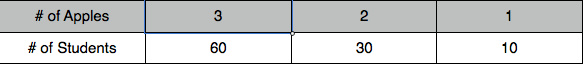What is the average number of apples a student has?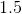Explanation:

To calculate the average number of apples a student has, the following formula is used.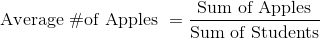First, calculate the total number of apples there are. To do this multiply the number of apples by the number of students that have that many apples.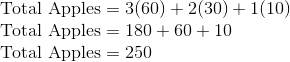This number divided by the total number of students.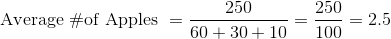### Example Question #1 : Statistics

The chart above lists the ages and heights of all the cousins in the Brenner family.  What is the average age of the female Brenner cousins?

17.1

19.3

16.4

18.7

16.2

16.2

Explanation:

There are five female cousins whose ages are 14, 22, 13, 12, and 20.

Add these up and divide by 5.

14 + 22 + 13 + 12 +20 = 81

81 / 5 = 16.2

### Example Question #1 : How To Find Arithmetic Mean

Find the arithmetic mean of the data set:

13, 21, 25, 37, 51, 52, 58, 83

42.5

70

83

13

44